Courses

# Digital Modulation Schemes GATE Notes | EduRev

## GATE : Digital Modulation Schemes GATE Notes | EduRev

The document Digital Modulation Schemes GATE Notes | EduRev is a part of the GATE Course Communication System.
All you need of GATE at this link: GATE

Digital Modulation Schemes

This is possible to transmit the analog signal i.e., speech, video etc, in digital format. Some digital modulation schemes are given below

• Digital Carrier Modulation: Commonly used digital modulation schemes are Amplitude Shift Keying (ASK), Frequency Shift Keying (FSK) and Phase Shift Keying (PSK).
• Amplitude Shift Keying (ASK): The amplitude of a high-frequency carrier is varied in accordance with digital data (0 or 1).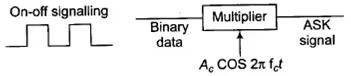S(t) = Ac cos 2πfct; 0 ≤ t ≤ Tb

= 0; otherwise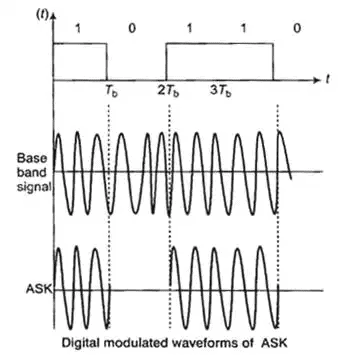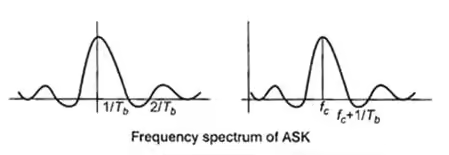Bandwidth = 2 × 1/Tb

= 2 × bit rate

• For digital input 1 amplitude level is high and for digital input 0 amplitude level is low.
• Signalling used is on-off signalling.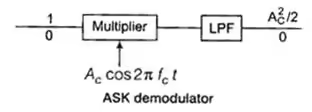For binary digit 1, Ac cos 2π fct × Ac cos 2πfct = (A2c /2)[1 + cos 4πfct]

Output of LPF = (A2/2)

For binary digit 0 output of LPF = 0

In ASK, probability of error (Pe) is high.

Phase Shift Keying (PSK):

In phase shift, keying phase of high-frequency carrier is varied in accordance with digital data 1 or 0.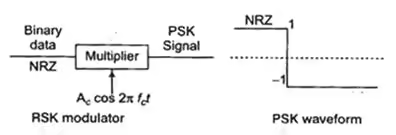• NRZ signalling is used.

S(t) = Ac cos 2πfct for bit 1

= – Ac cos 2pfct for bit 0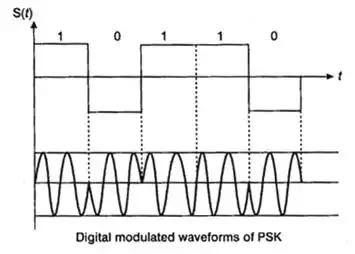A frequency of the carrier must be a multiple of a bit rate.

Tb = n/fc

Fc = nrb

• In case of PSK, a probability of error is less.
• In case of PSK, SNR is high.
• Mainly used a technique in wireless transmission.

Frequency Shift Keying (FSK):

In frequency shift keying, a frequency of the carrier is varied in accordance with digital data (1 or 0).

For digital data 1 we use frequency f1 and for digital data 0 we use frequency f2.• NRZ signalling is used here
• VCO The schematic diagram of VCO is given below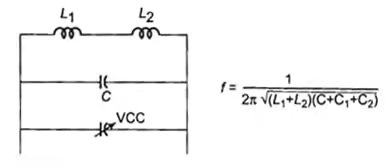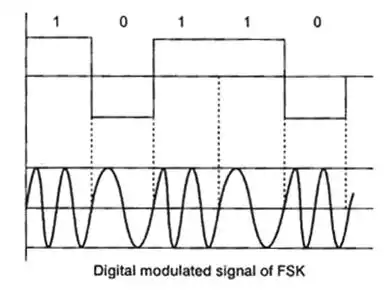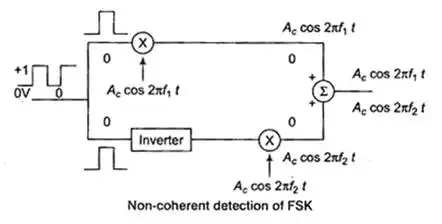Bandwidth = 2Δf + 2fm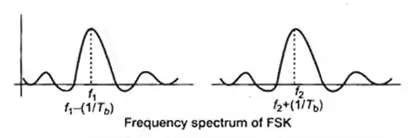Bandwidth = f1 + (1/Tb) – f2 + (1/Tb)

= f1 – f2 + (2/Tb); f1 – f2 = 2Δf

Key Points

• In case of FSK, Pe is less but SNR is high.
• Multiplexing is difficult in FSK.

Differential Phase Shift Keying (DPSK):

In PSK it needs a complicated synchronising circuit at the receiver, this disadvantage of PSK is removed in DPSK.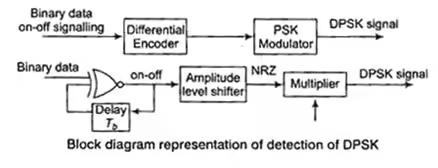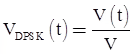A cos ω0t = ± A cos ω0t

Note: Advantage of DPSK over PSK is, DPSK does not require a coherent carrier for demodulation.

Comparison of Digital Modulation Schemes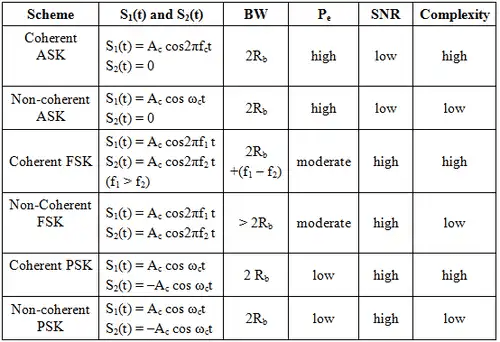In QAM, digital information is content in a both amplitude and phase of the signal. It is used in both digital modulation scheme and analog modulation scheme. Digital cable television and in cable modem applications QAM is used.

Offer running on EduRev: Apply code STAYHOME200 to get INR 200 off on our premium plan EduRev Infinity!

10 docs|12 tests

,

,

,

,

,

,

,

,

,

,

,

,

,

,

,

,

,

,

,

,

,

;